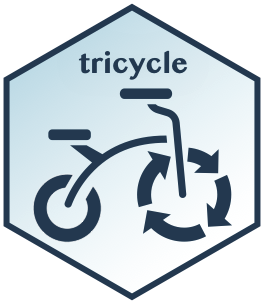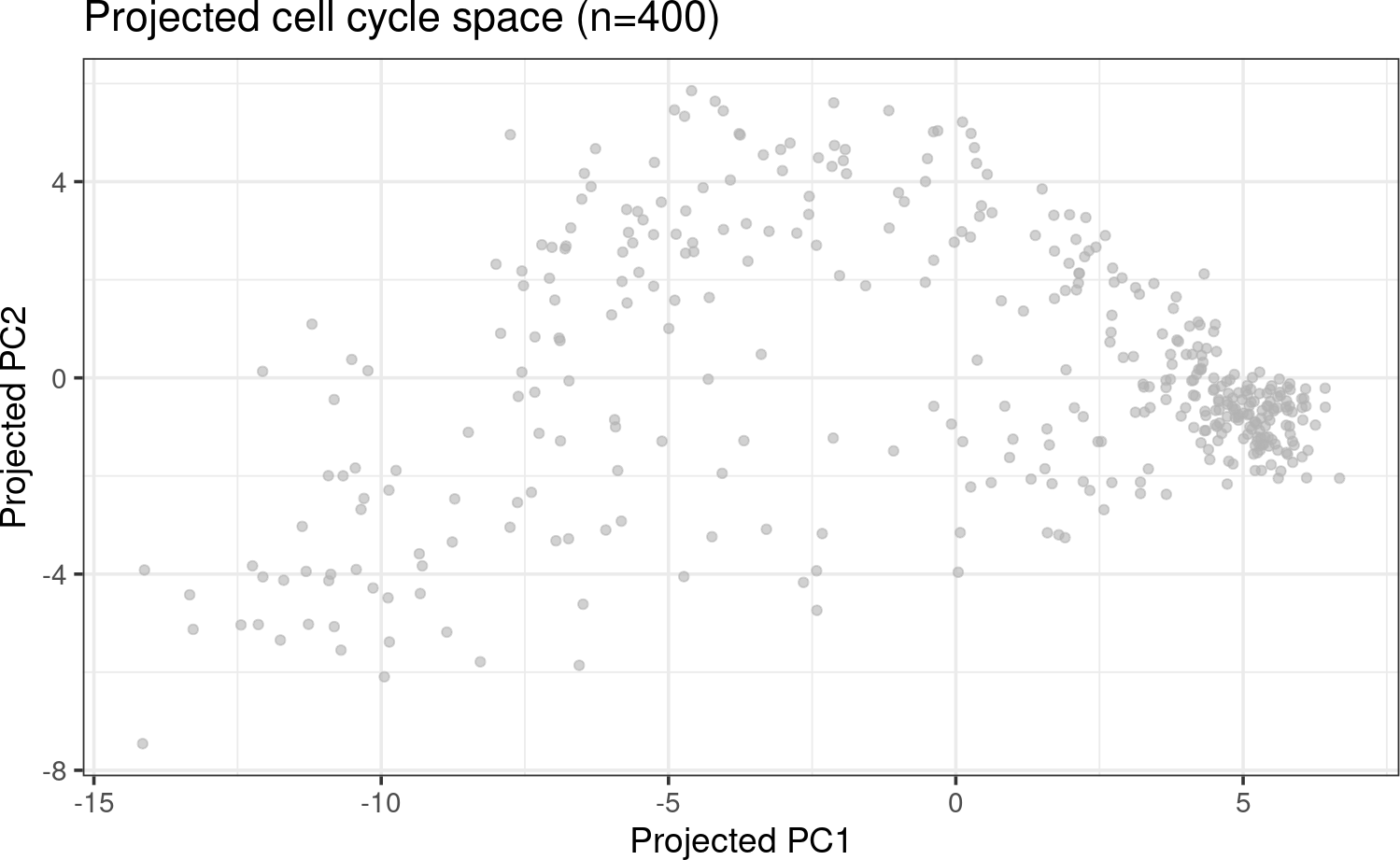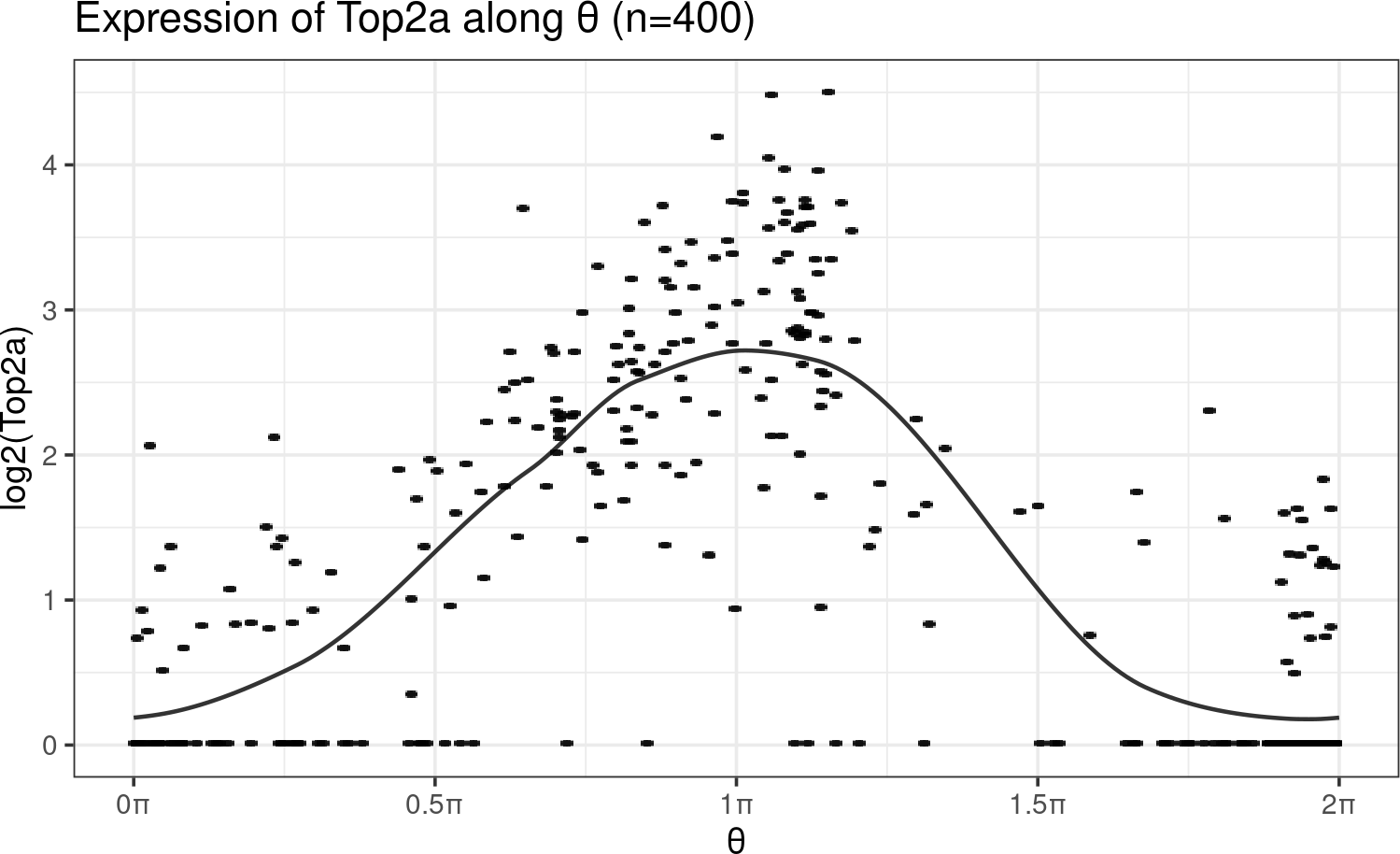# Contents# 1 Introduction

Here we describe a package for inferring cell cycle position for a single-cell RNA-seq dataset. The theoretical justification as well as benchmarks are included in (Zheng et al. 2022). In our hands, our approach (called TriCycle) works robustly across a variety of data modalities including across species (human and mouse), cell types and assay technology (10X, Fluidigm C1); we have yet to encounter a dataset where this approach does not work. The main output is a continuous estimate of the relative time within the cell cycle, represented as a number between 0 and 2pi (which we refer to as cell cycle position). In addition to the estimation process, we include a number of convenience functions for visualizing cell cycle time and we also provide an implementation of a discrete cell cycle stage predictor.

# 2 Prerequisites

library(tricycle)

We recommend users to start with a SingleCellExperiment object. The output will usually be the SingleCellExperiment with new info added. The functions work on matrix or SummarizedExperiment objects although the output changes, since these type of objects do not have the capability to store both the input object and the estimates.

In the package, we include a example SingleCellExperiment dataset, which is a real subset of mouse Neurosphere RNAseq of 2 samples. 200 cells from sample AX1 and AX2 were randonly sampled from the full data. This dataset is the same data as we use for constructing our cell cycle embedding.

data(neurosphere_example)

Important: Please note that the user should normalize library size before putting into the tricycle functions. The library size normalization could be done by normalizeCounts function in scater package or by calculating CPM values.

# 3 Overview of the package functionality

The method is based on taking a new dataset and projecting it into an embedding representing cell cycle. This embedding is constructed using a reference dataset. What is perhaps surprising is our finding that the same embedding is appropriate for all the experiments we have looked at, including across species, cell types and datasets. We are providing this embedding space as part of the package, and we do not expect users to change this embedding (although the functions in the package supports other embeddings).

The method is simple: you take a new dataset and project it into the latent space and infer cell cycle time. The key functions here are

• project_cycle_space()
• estimate_cycle_position()

The next step is to verify that the cell cycle time was successfully predicted. This involves looking at a number of useful plots. This involves a number of useful visualization. Note for example our use of color scheme - because cell cycle time “wraps around” the $$[0,2\pi]$$ interval, it is very useful to use a color palette which also “wraps around”. The relevant functions are

• plot_emb_circle_space()
• circle_space_legend()
• fit_periodic_loess()

We also provide a separate cell cycle stage predictor, predicting 5 different stages; estimate_Schwabe_stage(). This predictor is a small modification of the method proposed by (Schwabe et al. 2020). We include this function only for the purpose of convenience.
This is not part of tricycle method!

Finally we have a set of functions for creating your own reference latent space.

# 4 Project a single cell data set to pre-learned cell cycle space

project_cycle_space() will automatically project the assay with name logcounts into the cell cycle embedding without any other argument input. You could specify species (default as mouse), gene IDs, gene ID type, and AnnotationDb object if gene mapping is needed. Refer to man(project_cycle_space) for details.

neurosphere_example <- project_cycle_space(neurosphere_example)
#> No custom reference projection matrix provided. The ref learned from mouse Neuroshpere data will be used.
#> The number of projection genes found in the new data is 500.
neurosphere_example
#> class: SingleCellExperiment
#> dim: 1500 400
#> assays(2): counts logcounts
#> rownames(1500): ENSMUSG00000056763 ENSMUSG00000025925 ...
#>   ENSMUSG00000044221 ENSMUSG00000040234
#> rowData names(2): Gene Accession
#> colnames(400): AX1_CTACGTCCAAACCCAT AX1_AACTCTTTCATTCACT ...
#>   AX2_CGAGAAGCACTACAGT AX2_CATATTCGTCTGCGGT
#> colData names(2): TotalUMIs sample
#> reducedDimNames(1): tricycleEmbedding
#> mainExpName: NULL
#> altExpNames(0):

The projected cell cycle space will be stored in reducedDims with name “tricycleEmbedding” (you could set other embedding name.).

library(ggplot2)
library(scattermore)
library(scater)
scater::plotReducedDim(neurosphere_example, dimred = "tricycleEmbedding") +
labs(x = "Projected PC1", y = "Projected PC2") +
ggtitle(sprintf("Projected cell cycle space (n=%d)",
ncol(neurosphere_example))) +
theme_bw(base_size = 14)# 5 Infer cell cycle position

Once the new data has been projected into the cell cycle embedding, cell cycle position is estimated using estimate_cycle_position(). If the data has not been projected, this function will do the projection for you. Assuming a SingleCellExperiment as input, the cell cycle position will be addded to the colData of the object, with the name tricyclePosition.

neurosphere_example <- estimate_cycle_position(neurosphere_example)
names(colData(neurosphere_example))
#>  "TotalUMIs"        "sample"           "tricyclePosition"

The estimated cell cycle position is bound between 0 and 2pi. Note that we strive to get high resolution of cell cycle state, and we think the continuous position is more appropriate when describing the cell cycle. However, to help users understand the position variable, we also note that users can approximately relate 0.5pi to be the start of S stage, pi to be the start of G2M stage, 1.5pi to be the middle of M stage, and 1.75pi-0.25pi to be G1/G0 stage.

# 6 Assessing performance

We have two ways of (quickly) assessing whether TriCycle works. They are

1. Look at the projection of the data into the cell cycle embedding.
2. Look at the expression of key genes as a function of cell cycle position.

Plotting the projection of the data into the cell cycle embedding is shown above. Our observation is that deeper sequenced data will have a more clearly ellipsoid pattern with an empty interior. As sequencing depth decreases, the radius of the ellipsoid decreases until the empty interior disappears. So the absence of an interior does not mean the method does not work.

It is more important to inspect a couple of genes as a function of cell cycle position. We tend to use Top2a which is highly expressed and therefore “plottable” in every dataset. Other candidates are for example Smc2. To plot this data, we provide a convenient function fit_periodic_loess() to fit a loess line between the cyclic variable $$\theta$$ and other response variables. This fitting is done by making theta.v 3 periods (c(theta.v - 2 * pi, theta.v, theta.v + 2 * pi)) and repeating y 3 times. Only the fitted values corresponding to original theta.v will be returned. In this example, we show how well the expression of the cell cycle marker gene Top2a change along $$\theta$$.

top2a.idx <- which(rowData(neurosphere_example)$Gene == 'Top2a') fit.l <- fit_periodic_loess(neurosphere_example$tricyclePosition,
assay(neurosphere_example, 'logcounts')[top2a.idx,],
plot = TRUE,
x_lab = "Cell cycle position \u03b8", y_lab = "log2(Top2a)",
fig.title = paste0("Expression of Top2a along \u03b8 (n=",
ncol(neurosphere_example), ")"))
names(fit.l)
#>  "fitted"   "residual" "pred.df"  "loess.o"  "rsquared" "fig"
fit.l\$fig + theme_bw(base_size = 14)For Top2a we expect peak expression around $$\pi$$.

# 7 Alternative: Infer cell cycle stages

This method was proposed by Schwabe et al. (2020). We did small modifications to reduce NA assignments. But on average, the performance is quite similar to the original implementation in Revelio package. In brief, we calculate the z-scores of highly expressed stage specific cell cycle marker genes, and assgin the cell to the stage with the greatest z-score.

neurosphere_example <- estimate_Schwabe_stage(neurosphere_example,
gname.type = 'ENSEMBL',
species = 'mouse')
#> This function is a re-implementation of Schwabe et al. 2020. If you want to use tricycle method, please run estimate_cycle_position!
#> No gname input. Rownames of x will be used.
#>
#> No AnnotationDb desginated. org.Mm.eg.db will be used to map Mouse ENSEMBL id to gene SYMBOL.
#> Batch 1 phase G1.S gene:50
#> Batch 1 phase S gene:43
#> Batch 1 phase G2 gene:61
#> Batch 1 phase G2.M gene:67
#> Batch 1 phase M.G1 gene:30
scater::plotReducedDim(neurosphere_example, dimred = "tricycleEmbedding",
colour_by = "CCStage") +
labs(x = "Projected PC1", y = "Projected PC2",
title = paste0("Projected cell cycle space (n=", ncol(neurosphere_example), ")")) +
theme_bw(base_size = 14)